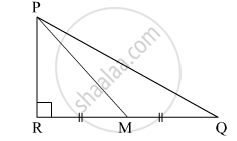# In the given figure, M is the midpoint of QR. ∠PRQ = 90°. Prove that, PQ2 = 4PM2 – 3PR2 - Geometry

Sum

In the given figure, M is the midpoint of QR. ∠PRQ = 90°. Prove that, PQ= 4PM– 3PR2#### Solution

According to Pythagoras theorem,

In ∆PRM,

PR2 + RM2 = PM2
⇒ RM2 = PM2 - PR2 ....(1)

In ∆PRQ,

PR2 + RQ2 = PQ2
⇒ PQ2 = PR2 + (RM + MQ)2
⇒ PQ2 = PR2 + (RM + RM)2
⇒ PQ2 = PR2 + (2RM)2
⇒ PQ2 = PR2 + 4RM2
⇒ PQ2 = PR2 + 4(PM2 - PR2) .....(from 1)
⇒ PQ2 = PR2 + 4PM2 - 4PR2
⇒ PQ2 = 4PM2 - 3PR2

Hence, PQ= 4PM– 3PR2.

Concept: Right-angled Triangles and Pythagoras Property
Is there an error in this question or solution?

#### APPEARS IN

Balbharati Mathematics 2 Geometry 10th Standard SSC Maharashtra State Board
Chapter 2 Pythagoras Theorem
Practice Set 2.1 | Q 9 | Page 39